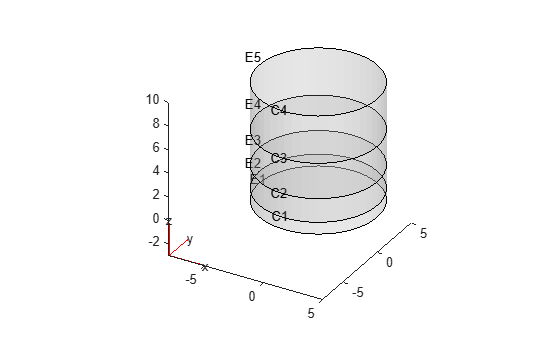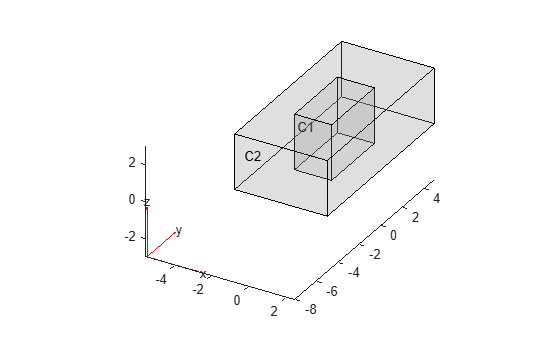# cellEdges

Find edges attached to specified cells

## Syntax

``EdgeID = cellEdges(g,RegionID)``
``EdgeID = cellEdges(g,RegionID,FilterType)``

## Description

example

````EdgeID = cellEdges(g,RegionID)` finds edges attached to the cells with ID numbers listed in `RegionID`.```

example

````EdgeID = cellEdges(g,RegionID,FilterType)` returns internal, external, or all edges attached to the cells with ID numbers listed in `RegionID`.```

## Examples

collapse all

Find edges attached to two middle cylinders in a geometry consisting of four stacked cylinders.

Create a geometry that consists of four stacked cylinders.

`gm = multicylinder(5,[1 2 3 4],'ZOffset',[0 1 3 6])`
```gm = DiscreteGeometry with properties: NumCells: 4 NumFaces: 9 NumEdges: 5 NumVertices: 5 Vertices: [5x3 double] ```

Plot the geometry with the cell and edge labels.

`pdegplot(gm,'CellLabels','on','EdgeLabels','on','FaceAlpha',0.2)`Find edges attached to cells 2 and 3.

`edgeIDs = cellEdges(gm,[2 3])`
```edgeIDs = 1×3 2 3 4 ```

Find edges attached to the outer cuboid in a geometry consisting of two nested cuboids.

Create a geometry that consists of two nested cuboids of the same height.

`gm = multicuboid([2 5],[4 10],3)`
```gm = DiscreteGeometry with properties: NumCells: 2 NumFaces: 12 NumEdges: 24 NumVertices: 16 Vertices: [16x3 double] ```

Plot the geometry with the cell labels.

`pdegplot(gm,'CellLabels','on','FaceAlpha',0.2)`Find all edges attached to the outer cell. Show the first 10 edges.

```edgeIDs = cellEdges(gm,2); edgeIDs(1:10)```
```ans = 1×10 1 2 3 4 5 6 7 8 9 10 ```

From all edges attached to the outer cell, return the edges attached to only the internal faces. Internal faces are faces shared between multiple cells.

`edgeIDs_int = cellEdges(gm,2,'internal')`
```edgeIDs_int = 1×4 9 10 11 12 ```

From all edges attached to the outer cell, return the edges attached to the external faces. Show the first 10 edges.

```edgeIDs_ext = cellEdges(gm,2,'external'); edgeIDs_ext(1:10)```
```ans = 1×10 1 2 3 4 5 6 7 8 13 14 ```

## Input Arguments

collapse all

3-D geometry, specified as a `DiscreteGeometry` object.

Cell ID, specified as a positive number or a vector of positive numbers. Each number represents a cell ID.

Type of edges to return, specified as `'internal'`, `'external'`, or `'all'`. Depending on this argument, `cellEdges` returns these types of faces:

• `'internal'` — Edges attached to only internal faces. Internal faces are faces shared between multiple cells.

• `'external'` — Edges attached to only external faces. External faces are faces not shared between multiple cells.

• `'all'` — All edges attached to the specified cells.

## Output Arguments

collapse all

IDs of edges attached to the specified cells, returned as a positive number or a vector of positive numbers.

## Version History

Introduced in R2021a